Next: Microscopic and Macroscopic Systems Up: Introduction Previous: What is Thermodynamics?

# Need for Statistical Approach

It is necessary to emphasize, from the very outset, that thermodynamics is a difficult subject. In fact, this subject is so difficult that we are forced to adopt a radically different approach to that employed in other areas of physics.

In most areas of physics, we can formulate some exact, or nearly exact, set of equations that governed the system under investigation. For instance, Newton's equations of motion, or Maxwell's equations for electromagnetic fields. We can then analyze the system by solving these equations, either exactly or approximately.

In thermodynamics, we have no problem formulating the governing equations. The motions of atoms and molecules are described exactly by the laws of quantum mechanics. In many cases, they are also described, to a reasonable approximation, by the much simpler laws of classical mechanics. We shall not be dealing with systems sufficiently energetic for atomic nuclei to be disrupted, so we need not consider nuclear forces. Also, in general, the gravitational forces between atoms and molecules are completely negligible. This implies that the forces between atoms and molecules are predominantly electromagnetic in origin, and are, therefore, very well understood. Thus, we can formulate the exact laws of motion for a thermodynamical system, including all of the inter-atomic forces. The problem is the sheer complexity of the resulting system of equations. In one mole of a substance (e.g., in twelve grams of carbon, or eighteen grams of water) there are Avagadro's number of atoms or molecules. That is, about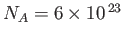particles. To solve the system exactly, we would have to write down approximately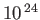coupled equations of motion, with the same number of initial conditions, and then try to integrate the system. This is plainly impossible. It would also be complete overkill. We are generally not interested in knowing the position and velocity of every particle in the system as a function of time. Instead, we want to know things like the volume of the system, the temperature, the pressure, the heat capacity, the coefficient of expansion, et cetera. We would certainly be hard put to specify more than about fifty, say, interesting properties of a thermodynamic system. In other words, the number of pieces of information that we require to characterize the state of the system is absolutely minuscule compared to the number of degrees of freedom of the system. That is, the number of pieces of information needed to completely specify its internal motion. Moreover, the quantities that we are interested in calculating do not depend on the motions of individual particles, or some some small subset of particles, but, instead, depend on the average motions of all the particles in the system. In other words, these quantities depend on the statistical properties of the atomic or molecular motion.

The method adopted in thermodynamics is essentially dictated by the enormous complexity of thermodynamic systems. We generally start off with some statistical information about the motions of the constituent atoms or molecules, such as their average kinetic energy, but possess very little information about the motions of individual particles. We then try to deduce some other properties of the system from a statistical treatment of the governing equations of motion. In fact, our approach has to be statistical in nature, because we lack most of the information required to specify the internal state of the system. The best we can do is to provide a few overall constraints, such as the average volume and the average energy.

Thermodynamic systems are ideally suited to a statistical approach, because of the enormous numbers of particles that they contain. Statistical arguments actually get more exact as the numbers involved get larger. For example, consider an opinion poll published in a newspaper. Such a poll usually specifies how many people were interviewed. This number is significant because, even if the polling was done without bias, which is extremely unlikely, the laws of statistics say that there is a intrinsic error (in the fraction of people who supposedly support a given opinion) of order one over the square root of the number of people questioned. It follows that if a thousand people were interviewed, which is a typical number, then the error is at least three percent. We can easily appreciate that if we do statistics on a thermodynamic system containingparticles then we are going to obtain results that are valid to incredible accuracy. In fact, in most situations, we can forget that the results are statistical at all, and treat them as exact laws of physics. For instance, the familiar equation of state of an ideal gas,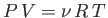(see Section 6.2), is actually a statistical result. In other words, it relates the average pressure, and the average volume, to the average temperature. However, for one mole of gas, the relative statistical deviations from average values are only about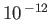, according to the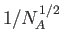law. Of course, it is virtually impossible to measure the pressure, volume, or temperature of a gas to such accuracy, so it is often convenient to forget about the fact that the ideal gas law is a statistical result, and treat it as a law of physics interrelating the actual pressure, volume, and temperature of an ideal gas.Next: Microscopic and Macroscopic Systems Up: Introduction Previous: What is Thermodynamics?
Richard Fitzpatrick 2016-01-25